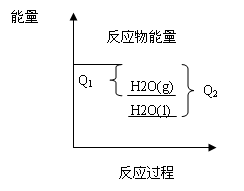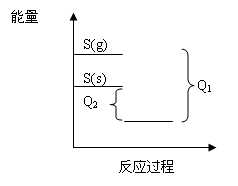# 化学自习室移动版

### 例谈反应热大小比较的两种方法

A.2H2(g)＋O2(g)=2H2O(g)；ΔH=－Q1

2H2(g)＋O2(g)=2H2O(l)；ΔH=－Q2

B.S(g)＋O2(g)=SO2(g)；ΔH=－Q1

S(S＋O2(g)=SO2(g)；ΔH=－Q2

C  C(s)＋0.5O2(g)=CO(g)；ΔH=－Q1

C(s)＋O2(g)=CO2(g)；ΔH=－Q2

D.H2(g)＋Cl2(g)=2HCl(g)；ΔH=－Q1

0.5H2(g)＋0.5Cl2(g)=HCl(g)；ΔH=－Q2

A项中两化学反应方程式反应物状态和系数都相同，只有产物状态不同，可得图像(如下图)B项中两化学反应方程式只有硫的状态不同，可得图像(如下图所示)A项中

①2H2(g)＋O2(g)=2H2O(g)；ΔH=－Q1

②2H2(g)＋O2(g)=2H2O(l)；ΔH=－Q2

①－②可得：2H2O(l)=2H2O(g)；ΔH=Q2－Q1

①S(g)＋O2(g)=SO2(g)；ΔH=－Q1

②S(s)＋O2(g)=SO2(g)；ΔH=－Q2

①－②可得：S(g)=S(s)H=ΔQ2－Q1

(责任编辑：)• 全部评论（0
还没有评论，快来抢沙发吧！
相关文章
作者其他文章
最近关注
热点内容
公益广告